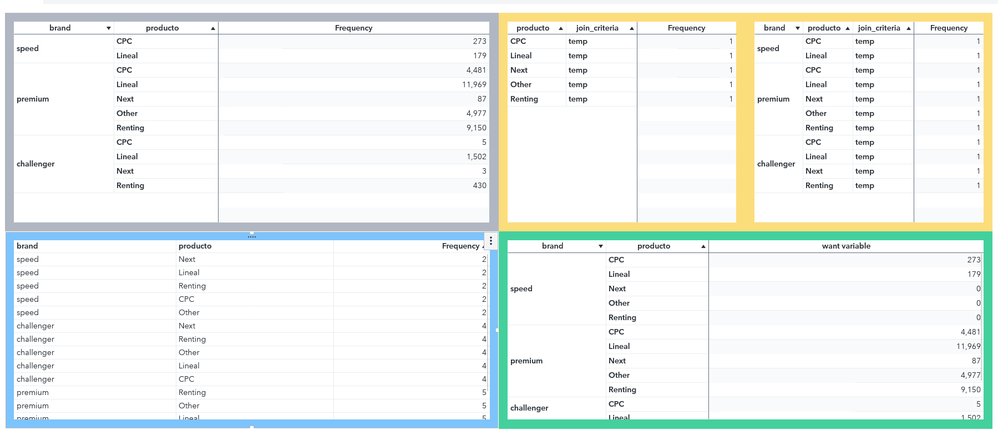## sas va key value object if no count how to display zero

Hi All,

I am using key value object and sector having the product count(measure) so it will display the name and count some sector does not have any count in that case sector name not appearing and also no count as zero.

## Re: sas va key value object if no count how to display zero

If the row does not exist, there's no direct way to solve it directly I guess.

BUT you could produce a cartesian product inside Visual Analytics by using the join option several times.

The grey cross table is the crossings in your table, the yellow ones are aggregated tables that are joined twice to produce the desired output (green).

The last joined table creates a calculated item if aggregated frequency is missing then 0, else aggregated frequency.here the BIRD code from the VA (ctrl + ALT + b)

``````       <DataSource label="ALL_MIGRATION_CT2_AGGREGATED (1)" type="relational" name="ds198">
<GeneratedResource type="standalone" resource="ge197" generator="dd194" source="ds7" lifetime="executor" externalReferenceName="cas-shared-defaultPublicALL_MIGRATION_CT2producto"/>
<GeneratedDataItem root="bi196" name="bi200" label="producto" xref="producto" usage="categorical" format="\$."/>
<PredefinedDataItem calculation="totalCount" name="bi201" label="Frequency" usage="quantitative" format="COMMA12."/>
<PredefinedDataItem calculation="totalCountPercent" name="bi202" label="Frequency Percent" usage="quantitative" format="PERCENT20.2"/>
<GeneratedDataItem root="bi214" name="bi217" label="join_criteria" xref="calculation" usage="categorical" format="\$."/>
</DataSource>
<DataSource label="ALL_MIGRATION_CT2_AGGREGATED" type="relational" name="ds185">
<GeneratedResource type="standalone" resource="ge175" generator="dd172" source="ds7" lifetime="executor" externalReferenceName="cas-shared-defaultPublicALL_MIGRATION_CT2productofrom_brand"/>
<GeneratedDataItem root="bi174" name="bi187" label="producto" xref="producto" usage="categorical" format="\$."/>
<GeneratedDataItem root="bi176" name="bi188" label="from_brand" xref="from_brand" usage="categorical" format="\$."/>
<GeneratedDataItem root="bi183" name="bi189" label="Aggregated Frequency" xref="calculation" usage="quantitative" format="COMMA12." aggregation="sum"/>
<PredefinedDataItem calculation="totalCount" name="bi190" label="Frequency" usage="quantitative" format="COMMA12."/>
<PredefinedDataItem calculation="totalCountPercent" name="bi191" label="Frequency Percent" usage="quantitative" format="PERCENT20.2"/>
<GeneratedDataItem root="bi208" name="bi211" label="join_criteria" xref="calculation1" usage="categorical" format="\$."/>
<GeneratedDataItem root="bi488" name="bi491" label="brand" xref="calculation2" usage="categorical" format="\$."/>
</DataSource>
<DataSource label="Data_Join (2)" type="relational" name="ds402">
<GeneratedResource type="standalone" resource="ge498" generator="dd495" lifetime="executor" sources="ds185 ds198" externalReferenceName="cas-shared-defaultPublicALL_MIGRATION_CT2calculation1from_brandcalculation2calculationproducto"/>
<GeneratedDataItem root="bi398" name="bi404" label="from_brand" xref="from_brand" usage="categorical" format="\$."/>
<GeneratedDataItem root="bi400" name="bi405" label="producto" xref="producto" usage="categorical" format="\$."/>
<PredefinedDataItem calculation="totalCount" name="bi406" label="Frequency" usage="quantitative" format="COMMA12."/>
<PredefinedDataItem calculation="totalCountPercent" name="bi407" label="Frequency Percent" usage="quantitative" format="PERCENT20.2"/>
<GeneratedDataItem root="bi501" name="bi506" label="brand" xref="calculation2" usage="categorical" format="\$."/>
</DataSource>
<DataSource label="Data_Join (3)" type="relational" name="ds429">
<GeneratedResource type="standalone" resource="ge513" generator="dd510" lifetime="executor" sources="ds402 ds185" externalReferenceName="cas-shared-defaultPublicALL_MIGRATION_CT2calculation2productofrom_brandcalculation2productocalculation"/>
<GeneratedDataItem root="bi423" name="bi431" label="from_brand" xref="from_brand" usage="categorical" format="\$."/>
<GeneratedDataItem root="bi424" name="bi432" label="producto" xref="producto" usage="categorical" format="\$."/>
<GeneratedDataItem root="bi427" name="bi433" label="Aggregated Frequency" xref="calculation" usage="quantitative" format="COMMA12." aggregation="average"/>
<PredefinedDataItem calculation="totalCount" name="bi434" label="Frequency" usage="quantitative" format="COMMA12."/>
<PredefinedDataItem calculation="totalCountPercent" name="bi435" label="Frequency Percent" usage="quantitative" format="PERCENT20.2"/>
<CalculatedItem dataType="double" name="bi479" label="want variable" usage="quantitative" format="COMMA12." aggregation="average">
<Expression>cond(ismissing(\${bi433,raw}),0,\${bi433,raw})</Expression>
</CalculatedItem>
<GeneratedDataItem root="bi514" name="bi522" label="brand" xref="calculation2" usage="categorical" format="\$."/>